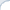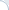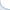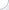English»Software»Machine Learning»Miscellany | searchivarius.orgCompression-based learning CudaMat   - Python module for performing basic dense linear algebra computations on the GPU using CUDA. cuDNN   - NVIDIA CUDA® Deep Neural Network library (cuDNN) is a GPU-accelerated library of primitives for deep neural networks. cuDNN provides highly tuned implementations for standard routines such as forward and backward convolution, pooling, normalization, and activation layers. cuDNN is part of the NVIDIA Deep Learning SDK. CUPY   - NumPy-like API accelerated with CUDA CuPy   - an open-source matrix library accelerated with NVIDIA CUDA (highly compatible with numpy). It also uses CUDA-related libraries including cuBLAS, cuDNN, cuRand, cuSolver and NCCL to make full use of the GPU architecture. JAX   - Autodiff and acceleration of numpy code. MiningMart project   - The basic idea is to store best practice cases of preprocessing chains that where developed by experienced users. The data is described on the meta level and is presented in application terms. MiningMart users choose a case and apply the corresponding transformation and learning chain to their application.preprocessing is the key issue in data analysis. Tensorly   - Tensor Learning in Python. The Uschificator is a method for scaling membership values of an argmax-classifier.   - The Uschificator is a method for scaling membership values of an argmax-classifier. A classifier is called argmax-classifier, if for ever class c ∈ C it computes a membership value m(x,c) which represents the classifiers certainty of the example x belonging to class c. Tpros and Cpros   - Gaussian Processes Papers and Software. This page contains two papers on Gaussian processes. The first paper (gpros.ps.gz) gives a full introduction to the use of Gaussian processes and a discussion of their application to regression problems. It also details approximate matrix inversion methods that can be used to implement Gaussian process regression efficiently. The second paper (class.ps.gz) describes how Gaussian processes can be applied to binary classification problems.